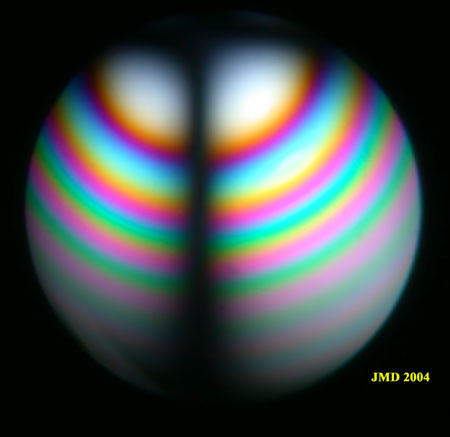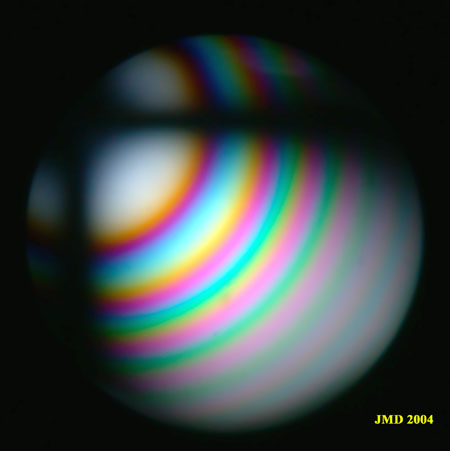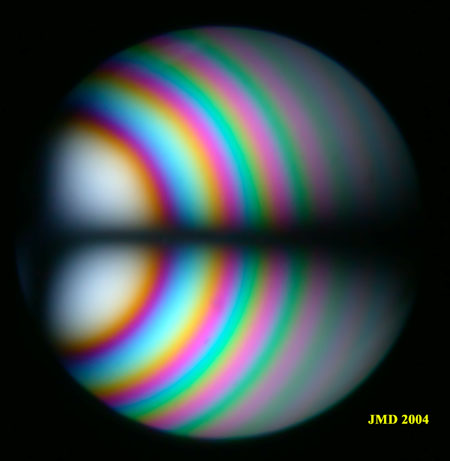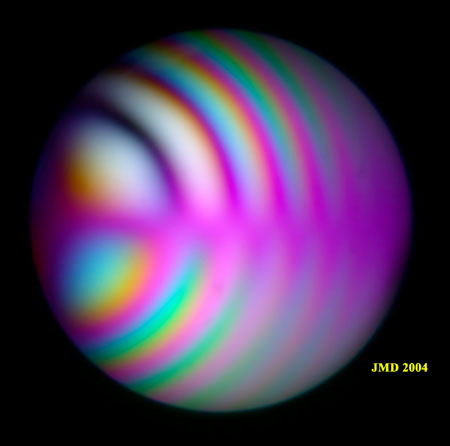# Beryl (Aqua Marine). Oblique section.

Oblique sections in uniaxial minerals can be easily recognized and used for the determination of the optic sign if the pole of the optic axis is not too far from the center of figure. In the example shown below, the pole of the optic axis is touching the circumference of the figure. If the microscope stage is rotated counterclockwise, the vertical isogyre of figure 1 is moving to the left, then the horizontal isogyre appears. This dark horizontal line is moving downwards on further rotation (see the sequence of figures 1,2 and 3). The addition of a wave plate to figure 3 allows the determination of the optical sign: there is a decrease of the retardation in the upper right quadrant (yellow color near the center and appearance of a zero order dark ring) so radial index is the smaller one and the crystal is negative.Figure 1. Oblique section in beryl.Figure 2. Same section rotated counterclockwise by an angle of 45°Figure 3. Further rotation  of 45°.Figure 4. Section shown in figure 3 with addition of a wave plate.

Video of the rotation of a thick section of beryl  not exactly perpendicular to the optic axis. This uniaxial crystal is slightly biaxial as can be seen by the splitting of the pole of the optic axis.